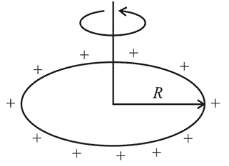# A thin ring of 10 cm radius carries a uniformly distributed charge.Question:

A thin ring of $10 \mathrm{~cm}$ radius carries a uniformly distributed charge. The ring rotates at a constant angular speed of $40 \pi \mathrm{rad} \mathrm{s}^{-1}$ about its axis, perpendicular to its plane. If the magnetic field at its centre is $3.8 \times 10^{-9} \mathrm{~T}$, then the charge carried by the ring is close to $\left(\mu_{0}=4 \pi \times 10^{-7} \mathrm{~N} / \mathrm{A}^{2}\right)$.

1. (1) $2 \times 10^{-6} \mathrm{C}$

2. (2) $3 \times 10^{-5} \mathrm{C}$

3. (3) $4 \times 10^{-5} \mathrm{C}$

4. (4) $7 \times 10^{-6} \mathrm{C}$

Correct Option: , 2

Solution:

(2) If $q$ is the charge on the ring, then$i=\frac{q}{T}=\frac{q \omega}{2 \pi}$

Magnetic field,

$B=\frac{\mu_{0} i}{2 R}$

$=\frac{\mu_{0}\left(\frac{q \omega}{2 \pi}\right)}{2 R}$

or $3.8 \times 10^{-9}=\left(\frac{\mu_{0}}{4 \pi}\right) \frac{q \omega}{R}=\left(10^{-7}\right) \frac{q \times 40 \pi}{0.10}$

$\therefore q=3 \times 10^{-5} \mathrm{C}$.